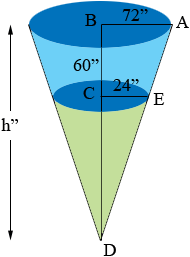SEARCH HOMEMath Central Quandaries & QueriesQuestion from Dianna: I need to figure the cubic yards of a cyclone. 144" down to 48" 60" highHi Dianna,

I am going to assume that the cyclone is approximated by a truncated cone, that is a cone with the bottom cut off as in the diagramThe volume of a cone is 1/3 π r2 h where r is the radius and h is the height. The volume of the truncated cone is then the difference between the volume of the large cone and the volume of the smaller cone shaded green. You know the radii of each of the cones and I called the height of the large cone h inches. The height of the smaller cone is h - 60 inches and hence if you can find the value of h you can calculate the volume of the truncated cone. Since your units are inches this will give the volume in cubic inches. If you want the volume in cubic yards I would divide each of the lengths by 36 to convert the lengths to yards, and then perform the calculations.

You can find the length h using similar triangles. Triangles ABD and ECD are similar so

|AB|/|BD| = |EC|/|CD|

or

72/h = 24/(h - 60).

Solve for h.

PennyMath Central is supported by the University of Regina and The Pacific Institute for the Mathematical Sciences.